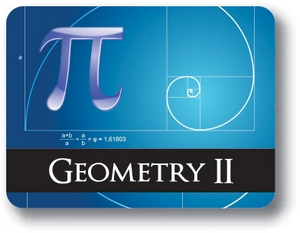# Geometry Semester 2• Course Credits: 0.5
• Course Price: \$0.00

## Course Overview:

This course provides an opportunity for students to further explore geometric relationships. Students perform constructions, explore relationships among geometric figures, formulate and prove geometric conjectures. Topics include the study of similar figures and the use of these concepts to introduce trigonometric relationships. The properties of quadrilaterals and circles are also studied. The connection between 2-dimensional figures and 3-dimensional solids are explored and analyzed. The student will explore the concepts of compound and conditional probability.

Note: This course is not designed for ELL (English Language Learners) students. ELL students may enroll in this course ONLY if they have adequate mentor support at their home school and are able to fulfill all course requirements.

## Prerequisites:

Materials: Students will use an online program called Geogebra for many activities and will also need a scientific calculator.

## Syllabus:

Section 1 - Similarity

Objectives:

Explore the relationship between dilation and similarity; Apply the concepts of similarity to understand the trigonometric ratios; Use Trigonometric concepts to solve problems; Prove theorems about similar figures and proportions in triangles.

Lessons:

• Dilations & Similarity
• Triangle Similarity
• Proportionality Theorems
• Formulate proofs of theorems
• Geogebra : Dilation Activity
• Geogebra: AA Similarity Activity
• Geogebra: Proportional Activities (3)

Section 2 – Right Triangle Trigonometry

Objectives:

Understand the relationship between similar triangles and trigonometry; Use the geometric mean to solve problems; Solve right triangles using trig ratios, Use the Law of sines and cosines to solve all triangles; Solve real world problems.

Lessons:

• Similarity in right triangles
• Trig ratios
• Solve right triangles
• Law of sines and cosines
• Apply trig concepts to solve real world problems

Objectives:

State the properties of a parallelogram; Prove parallelogram theorems; Determine if geometric figures are parallelograms; Classify figures in the coordinate plane; Prove the slope criteria for parallel lines.

Lessons:

• Parallelograms
• Geometry in the coordinate plane
• Construction: Square

Section 4 – Exploring Circles

Objectives:

Derive the equation of a circle by completing the square; prove theorems about circles; Calculate the area of a sector and arc length; Find the measures of inscribed angles; Find unknown segment lengths.

Lessons:

• Introduction to circles
• Arcs of a circle
• Angle relationships in circles
• Segment relationships in circles
• Construction: Regular polygon inscribed in a circle

Section 5 - Geometric Measurements

Objectives:

Calculate perimeter and area of polygons on the coordinate plane; Identify 2D cross sections of 3D objects; Identify 3D objects generated by rotating 2D figures; Derive volume formulas using Cavalieri’s Principle, dissection and reasoning; Calculate the volume of spheres, pyramids, cylinders and cones; Calculate the volume of similar figures. Use geometric modeling to solve real world problems.

Lessons:

• Measurements on the coordinate plane
• Exploring 3D figures
• Volume of geometric solids
• Geometric modeling

Section 6 - Probability

Objectives:

Calculate experimental and theoretical probability. Make and use frequency tables. Use permutation and combinations to solve problems. Find compound and conditional probabilities. Create and use simulations to model real world problems.

Lessons:

• Introduction to probability
• Permutations & combinations
• Compound probability
• Conditional probability
• Modeling with simulations

## Awards, Approvals, and Accreditation

••••••••••••••••••